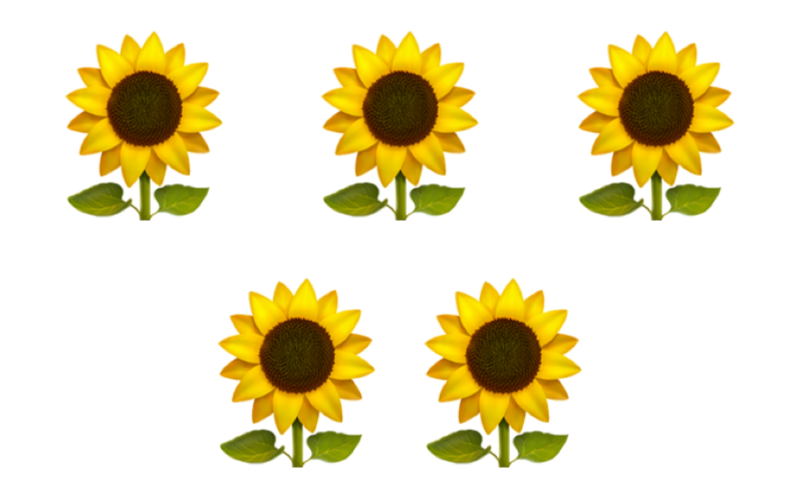maths > integers

Directed Numbers

what you'll learn...

overview

Considering a person receiving $3$$3$ candies and given $3$$3$ candies. These two are represented as $3$$3$ in whole numbers. But, received $3$$3$ and given $3$$3$ are two different quantities.

It is noted that received $3$$3$ and given $3$$3$ together make $3-3=0$$3 - 3 = 0$. So minus sign is adopted to provide the missing information in the whole numbers.

Taking "receiving" as the direction of reference, the integers are defined as

•  $\text{received:}3=+3$$\textrm{\left(r e c e i v e d\right\rangle} 3 = + 3$

•  $\text{given:}3=-3$$\textrm{\left(g i v e n\right\rangle} 3 = - 3$

give 2 or take 2The number of flowers in the picture is 5.

This is an example of using a whole number to count or measure quantities.

Some early humans naturally used hands to count similar to the numbers $1,2,3,\cdots$$1 , 2 , 3 , \cdots$. Natural numbers or Counting numbers are $1,2,3,\cdots$$1 , 2 , 3 , \cdots$

Whole numbers are $0$$0$ along with natural numbers.

Let us recall what is a whole number.

A whole number is a count or measurement of a quantity.

•  Number of pencils is $20$$20$.

•  Length of a pencil is $14$$14$cm

•  Mass of a iron ball is $2$$2$kg

•  Volume of a bottle of water is $1$$1$ liter

•  Time taken to finish a work is $30$$30$mins

•  Temperature of water is ${26}^{\circ }$${26}^{\circ}$C

•  Price of a pen is $15$$15$ coins

Consider a girl and her brother sharing candies. She receives $2$$2$ candies from her brother. The number of candies received is $2$$2$.

On another day, The girl gives $2$$2$ candies to her brother. The number of candies given is $2$$2$.

Considering whole numbers only. A girl received $2$$2$ candies from her brother. This is represented by the number $2$$2$.

On another day, she gives $2$$2$ candies to her brother. This is represented by the number $2$$2$.

Note that both the numbers are represented as $2$$2$. Are these two quantities the same?

Though the values of the numbers are same, the number 2 received is different from 2 given.

Consider the two situations.
A girl receives $2$$2$ candies from her father. On another day, she receives another $2$$2$ candies from her father. Effectively, she has received, $2+2=4$$2 + 2 = 4$ candies from her father.

The girl receives $2$$2$ candies from her brother. On another day, she gives $2$$2$ candies to her brother. Overall, she has received $2$$2$ candies and returned $2$$2$ candies. This makes total of, $2-2=0$$2 - 2 = 0$ candies received from her brother.

It is noted that the $2$$2$ received and $2$$2$ given are different quantities.

$2$$2$ received is aligned to the direction of receiving. And $2$$2$ given is opposed to the direction of receiving. They together make $0$$0$.

A girl receives $10$$10$ candies from her brother. Later, she gives $2$$2$ candies to her brother.

She has $10-2=8$$10 - 2 = 8$ candies.

It is noted that the given is subtracted.

The two inherent properties of the numbers

•  The quantities received and given represent two different values.

•  When combined, the quantities received and given are subtracted.

In accordance with these, negative numbers are introduced. Minus sign is adopted to provide the missing information in the numbers.

For Example:

$2$$2$ received is $+2$$+ 2$ or $2$$2$

$2$$2$ given is $-2$$- 2$

directed numbers

The count of candies is understood in two different forms

•  $5$$5$ candies received.

•  $5$$5$ candies given.

Similarly other quantities have two different forms.

•  Height above and below ground level

•  Distance to reach a city and distance covered after crossing the city

•  Length or Mass or Volume : amount available to use and amount used in excess

•  Temperature above ${0}^{\circ }$${0}^{\circ}$C or below ${0}^{\circ }$${0}^{\circ}$C

•  Temperature to heat or cool down

•  Time remaining or time passed since start

•  Money credited or debited

All these applications are modeled with numbers given in the form
$3$$3$ and $-3$$- 3$
which are equivalently given as $\text{received:}3=3$$\textrm{\left(r e c e i v e d\right\rangle} 3 = 3$ and $\text{given:}3=-3$$\textrm{\left(g i v e n\right\rangle} 3 = - 3$ to explain some fundamentals in arithmetics.

Such numbers with positive and negative direction are called Directed Numbers.

Integers are directed whole numbers.

terminology

The word "negative" means: absence of something; not having or does not exist.

The word "positive" means: presence of something; having or existing.

The word "directed" means: aimed in a particular direction.

The positive numbers represent numbers aligned to a direction and negative numbers represent numbers opposed to the direction.

Integers : Directed whole numbers are called integers.

example

If $2$$2$ degree above $0$$0$ is $+2$$+ 2$, what is $7$$7$ degree below $0$$0$?
The answer is '$-7$$- 7$'.

summary

Integers : Directed whole numbers are called integers.

Positive Integers : Numbers that are aligned to the chosen direction are positive integers and are given with $+$$+$ symbol or without that.
eg: $+2$$+ 2$ or $2$$2$.

Negative Integers : Numbers that are opposed to the chosen direction are negative integers and are given with $-$$-$ symbol.
eg: $-2$$- 2$

Integer form $2$$2$ is $\text{received:}2$$\textrm{\left(r e c e i v e d\right\rangle} 2$ in directed whole number form. Integer form $-2$$- 2$ is $\text{given:}2$$\textrm{\left(g i v e n\right\rangle} 2$ in directed whole number form.

Outline